# Pressure equation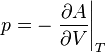$p = -\left. \frac{\partial A}{\partial V}\right\vert_T$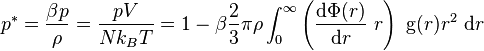$p^*=\frac{\beta p}{\rho}= \frac{pV}{Nk_BT} = 1 - \beta \frac{2}{3} \pi \rho \int_0^{\infty} \left( \frac{{\rm d}\Phi(r)} {{\rm d}r}~r \right)~{\rm g}(r)r^2~{\rm d}r$
where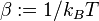$\beta := 1/k_BT$,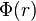$\Phi(r)$ is a central potential and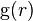${\rm g}(r)$ is the pair distribution function.